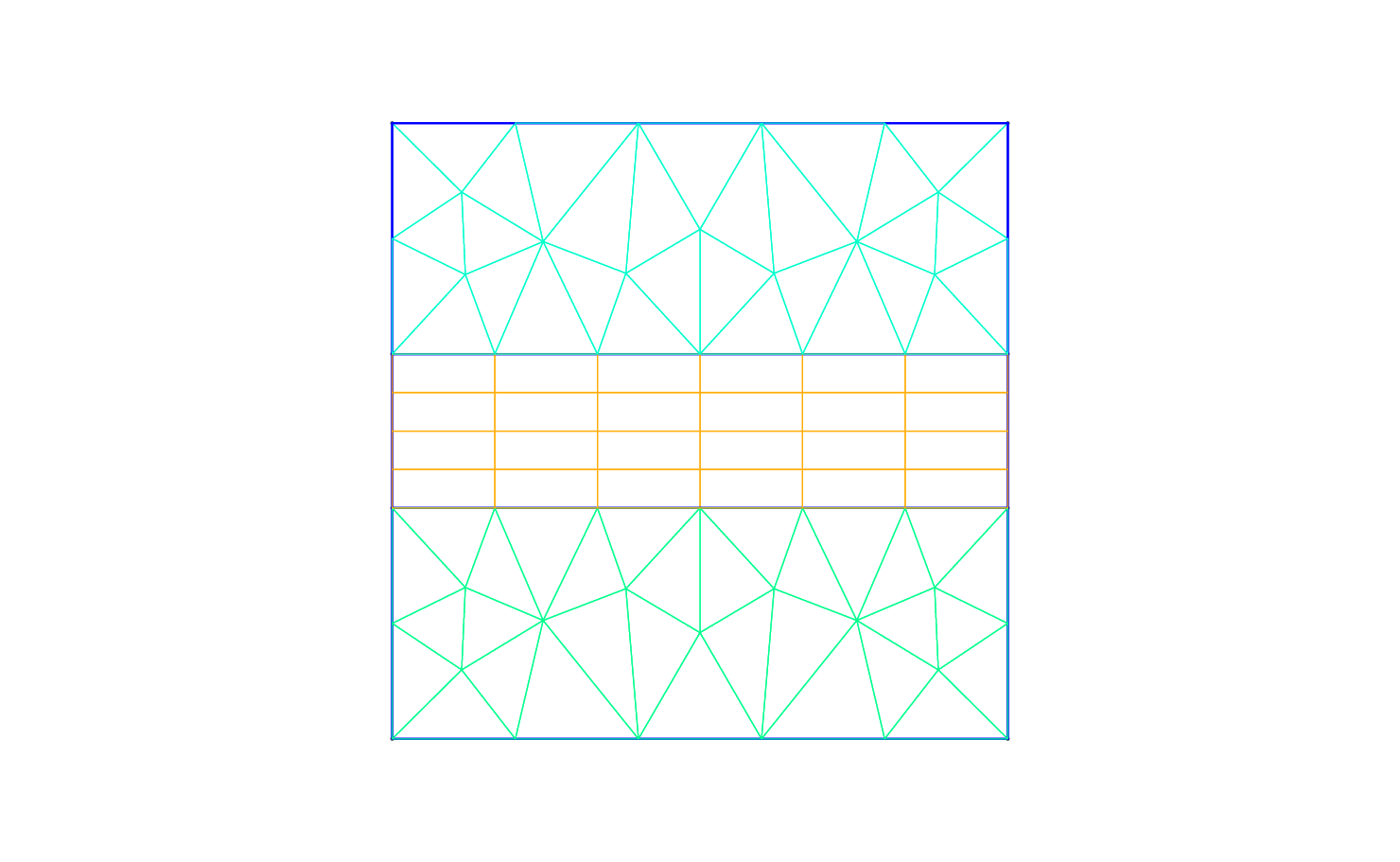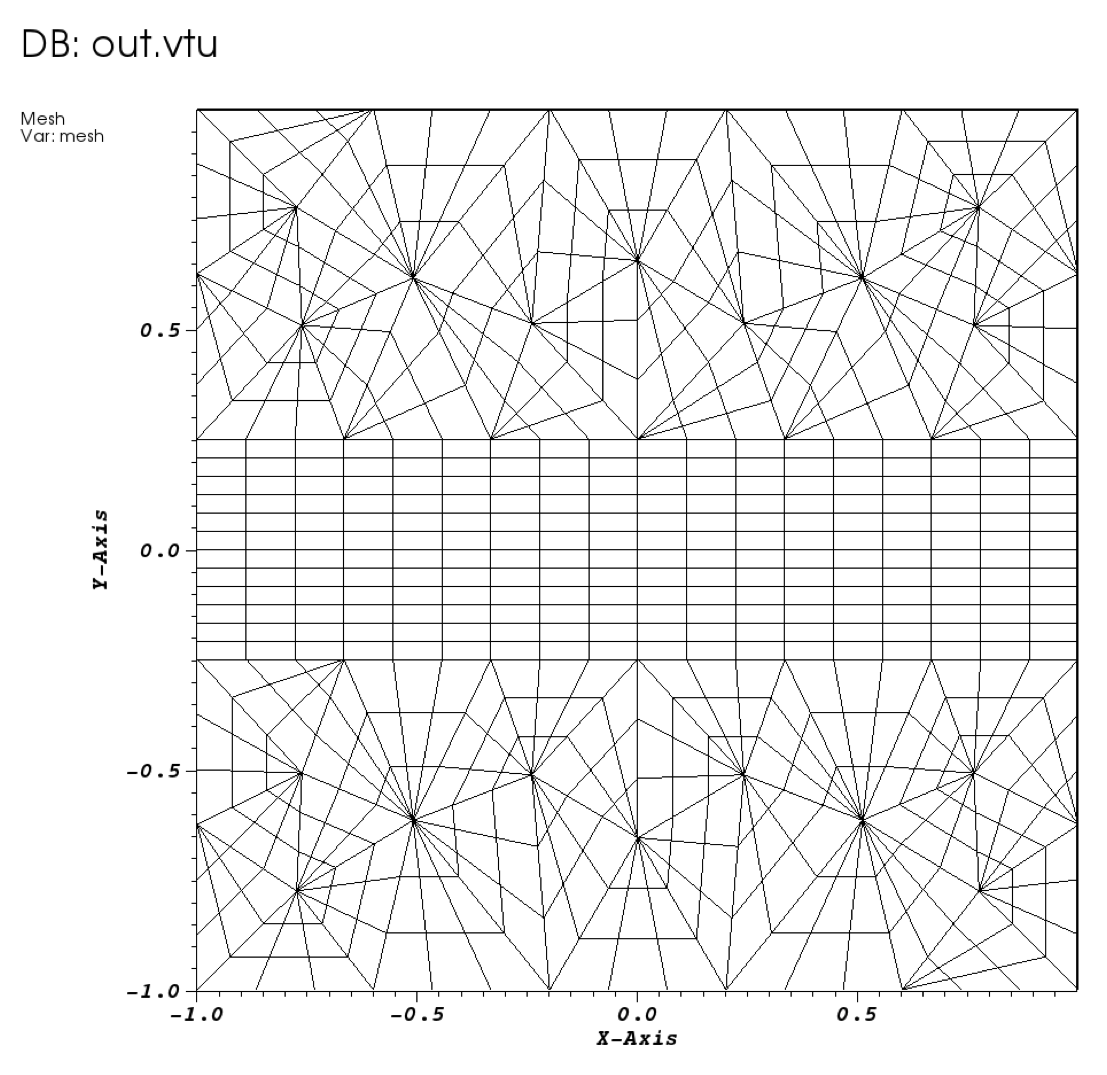## Chapter 2Pre-processing

The pre-processing step consists of generating the mesh in a Nektar++ compatible format. To do this we can use the open-source mesh generator Gmsh to first define the geometry, which in our case is a square mesh. The resulting mesh is shown in Fig. 2.1. The mesh file format (.msh) generated by Gmsh is not directly compatible with the Nektar++ solvers and, therefore, it needs to be converted.Figure 2.1: Mesh generated by Gmsh.

To do so, we need to run the Nektar++ pre-processing routine called `NekMesh`. This routine requires two command-line arguments: the mesh file generated by Gmsh, `Helm_mesh.msh`; and the name of the Nektar++-compatible mesh file that `NekMesh` will generate, for instance `Helm_mesh.xml`.

Task: 2.1 Convert the .msh file into a Nektar++ input from within the `\$NEKTUTORIAL` directory by calling

`\$NEK/NekMesh Helm_mesh.msh Helm_mesh.xml`

or

`\$NEK/NekMesh Helm_mesh.msh Helm_mesh.xml:xml:uncompress`

Note that by default the information is stored in XML blocks of compressed data to reduce the size of large meshes. The second command above tells the converter `NekMesh` to write the file out in uncompressed ASCII format. The generated .xml mesh file is shown below and can also be found in the `completed` directory. It contains 5 tags encapsulated within the `GEOMETRY` tag, which describes the mesh. The first tag, `VERTEX`, contains the spatial coordinates of the vertices of the various elements of the mesh. The second tag, `EDGE` contains the lines connecting the vertices. The third tag, `ELEMENT`, defines the elements (note that in this case we have both triangular - e.g. `<T ID="0">` - as well as quadrilateral - e.g. ```<Q ID="85">``` - elements). The fourth tag, `COMPOSITE`, describes the physical regions of the mesh and each composite may contain only one type of mesh entity. There are three composites composed of triangular or quadrilateral elements which represent the solution sub-domains where we want to solve the linear advection problem. We will use these composites to define expansion bases on each sub-domain in section 2.1. The composites formed by edges are the boundaries of the domain where we will prescribe suitable boundary conditions in section 2.1. Finally, the fifth tag, `DOMAIN`, formally specifies the overall solution domain as the union of the three element composites. For additional details on the `GEOMETRY` specification refer to the User-Guide.

1<?xml version="1.0" encoding="utf-8" ?>
2<NEKTAR>
3   <GEOMETRY DIM="2" SPACE="2">
4      <VERTEX>
5        <V ID="0">2.00000000e-01 -1.00000000e+00 0.00000000e+00</V>
6        <V ID="1">5.09667784e-01 -6.15240515e-01 0.00000000e+00</V>
7        ...
8        <V ID="68">-1.00000000e+00 1.25000000e-01 0.00000000e+00</V>
9      </VERTEX>
10      <EDGE>
11        <E ID="0"> 0 1 </E>
12        <E ID="1"> 1 2 </E>
13        ...
14        <E ID="153"> 40 68 </E>
15      </EDGE>
16      <ELEMENT>
17        <T ID="0"> 0 1 2 </T>
18        <T ID="1"> 3 4 5 </T>
19        ...
20        <Q ID="85"> 146 93 153 151 </Q>
21     </ELEMENT>
22      <COMPOSITE>
23        <C ID="1"> T[0-30] </C>
24        <C ID="2"> Q[62-85] </C>
25        <C ID="3"> T[31-61] </C>
26        <C ID="100"> E[46,12,20,10,45] </C>
27        <C ID="200"> E[50,32,108,111,114,117,87,103] </C>
28        <C ID="300"> E[100,64,74,66,99] </C>
29        <C ID="400"> E[49,33,148,150,152-153,86,104] </C>
30      </COMPOSITE>
31      <DOMAIN> C[1,2,3] </DOMAIN>
32   </GEOMETRY>
33</NEKTAR>

After generating the mesh file in the Nektar++-compatible format, `Helm_mesh.xml`, we can visualise the mesh. This step can be done by using the following Nektar++ built-in post-processing routine:

Task: 2.2 Convert the .xml file into a .vtu format within the `\$NEKTUTORIAL` directory by calling

`\$NEK/FieldConvert Helm_mesh.xml Helm_mesh.vtu`

Alternatively a tecplot .dat file can be created by changing the extension of the second file, i.e.

`\$NEK/FieldConvert Helm_mesh.xml Helm_mesh.dat`

This will produce a `Helm_mesh.vtu` file which can be directly read by the open-source visualisation tool called Paraview or VisIt. In Fig. 2.2 we show the mesh distribution for the mesh considered in this tutorial, `Helm_mesh.xml`.Figure 2.2: Mesh distribution with local polynomial subdivision

We can now configure the conditions: initial conditions, boundary conditions, parameters and solver settings.

### 2.1 Configuring the expansion bases and the conditions

To set the various parameters, the solver settings and the initial and boundary conditions needed, we create a new file called `Helm_conditions.xml`, which can be found within the `completed` directory for this tutorial. This new file contains the `CONDITIONS` tag where we can specify the parameters of the simulations, the solver settings, the initial conditions, the boundary conditions and the exact solution and contains the `EXPANSIONS` tag where we can specify the polynomial order to be used inside each element of the mesh, the type of expansion bases and the type of points.

We begin to describe the `Helm_conditions.xml` file from the `CONDITIONS` tag, and in particular from the boundary conditions, initial conditions and exact solution sections:

1<CONDITIONS>
2   ...
3   ...
4   ...
5   <VARIABLES>
6      <V ID="0"> u </V>
7   </VARIABLES>
8
9   <BOUNDARYREGIONS>
10      <B ID="0"> C </B>
11      <B ID="1"> C </B>
12      <B ID="2"> C </B>
13      <B ID="3"> C </B>
14   </BOUNDARYREGIONS>
15
16   <BOUNDARYCONDITIONS>
17      <REGION REF="0">
18        <N VAR="u" VALUE="-PI*cos(PI*x)*sin(PI*y)" />
19      </REGION>
20      <REGION REF="1">
21        <D VAR="u" VALUE="cos(PI*x)*cos(PI*y)" />
22      </REGION>
23      <REGION REF="2">
24        <N VAR="u" VALUE="PI*cos(PI*x)*sin(PI*y)" />
25      </REGION>
26      <REGION REF="3">
27        <D VAR="u" VALUE="cos(PI*x)*cos(PI*y)" />
28      </REGION>
29   </BOUNDARYCONDITIONS>
30
31   <FUNCTION NAME="Forcing">
32      <E VAR="u" VALUE="-(Lambda␣+␣2*PI*PI)*cos(PI*x)*cos(PI*y)" />
33   </FUNCTION>
34
35   <FUNCTION NAME="ExactSolution">
36      <E VAR="u" VALUE="cos(PI*x)*cos(PI*y)" />
37   </FUNCTION>
38</CONDITIONS>

In the above piece of `.xml`, we first need to specify the non-optional tag called `VARIABLES` that sets the solution variable (in this case u).

The second tag that needs to be specified is `BOUNDARYREGIONS` through which the user can specify the regions where to apply the boundary conditions. For instance, ```<B ID="0"> C </B>``` indicates that composite 100 (which has been introduced in section 2) has a boundary ID equal to 0. This boundary ID is successively used to prescribe the boundary conditions.

The third tag is `BOUNDARYCONDITIONS` by which the boundary conditions are actually specified for each boundary ID specified in the `BOUNDARYREGIONS` tag. The syntax `<D VAR="u"` corresponds to a `D`irichlet boundary condition for the variable `u`, while `<N VAR="u"` corresponds to `N`eumann boundary conditions. For additional details on the various options possible in terms of boundary conditions refer to the User-Guide.

Finally, `<FUNCTION NAME="Forcing">` allows the specification of the Forcing function and `<FUNCTION NAME="ExactSolution">` permits us to provide the exact solution, against which the L2 and L errors are computed.

After having configured the `VARIABLES` tag, the boundary conditions, the forcing function and the exact solution we can complete the tag `CONDITIONS` prescribing the parameters necessary (`PARAMETERS`)and the solver settings (`SOLVERINFO`):

1<CONDITIONS>
2   <PARAMETERS>
3      <P> Lambda= 2.5 </P>
4   </PARAMETERS>
5
6   <SOLVERINFO>
7      <I PROPERTY="EQTYPE" VALUE="Helmholtz" />
8      <I PROPERTY="Projection" VALUE="Continuous" />
9   </SOLVERINFO>
10   ...
11   ...
12   ...

In the `PARAMETERS` tag, `Lambda` is the Helmholtz constant λ.

In the `SOLVERINFO` tag, `EQTYPE` is the type of equation to be solved, `Projection` is the spatial projection operator to be used (which in this case is specified to be ‘Continuous’) For additional solver-setting options refer to the User-Guide.

Finally, we need to specify the expansion bases we want to use in each of the three composites or sub-domains (`COMPOSITE=".."`) introduced in section 2:

1<EXPANSIONS>
2   <E COMPOSITE="C" NUMMODES="5" TYPE="MODIFIED" FIELDS="u" />
3   <E COMPOSITE="C" NUMMODES="5" TYPE="MODIFIED" FIELDS="u" />
4   <E COMPOSITE="C" NUMMODES="5" TYPE="MODIFIED" FIELDS="u" />
5</EXPANSIONS>

In particular, for all the composites, `COMPOSITE="C[i]"` with i=1,2,3 we select identical basis functions and polynomial order, where `NUMMODES` is the number of coefficients we want to use for the basis functions (that is commonly equal to P+1 where P is the polynomial order of the basis functions), `TYPE` allows selecting the basis functions `FIELDS` is the solution variable of our problem and `COMPOSITE` are the mesh regions created by Gmsh. For additional details on the `EXPANSIONS` tag refer to the User-Guide.

Task: 2.3 Generate the file `Helm_conditions.xml` in the directory `\$NEKTUTORIAL` with λ = 2.5 or copy it from the `completed` directory.